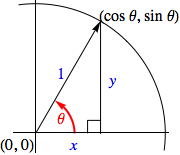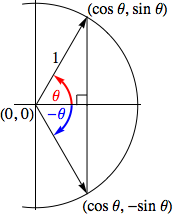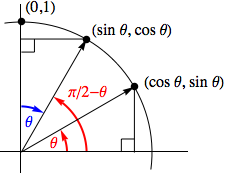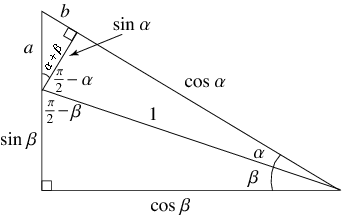## Some Useful Trigonometric Identities

An identity is an equation whose left and right sides -- when defined -- are always equal regardless of the values of the variables the two sides contain.

Some very useful trigonometric identities are shown below.

The Pythagorean Identities

$$\begin{array}{c} \cos^2 \theta + \sin^2 \theta = 1\\ 1 + \tan^2 \theta = \sec^2 \theta\\ 1 + \cot^2 \theta = \csc^2 \theta \end{array}$$

Even/Odd Function Identities

$$\begin{array}{rcl} \cos (-\theta) &=& \phantom{-}\cos \theta\\ \sin (-\theta) &=& -\sin \theta\\ \tan (-\theta) &=& -\tan \theta \\ \end{array}$$

Complementary Angle Identities

$$\begin{array}{rcl} \cos(\pi/2 - \theta) &=& \sin \theta\\ \sin(\pi/2 - \theta) &=& \cos \theta\\ \tan(\pi/2 - \theta) &=& \cot \theta \end{array}$$

Sum and Difference Identities

$$\begin{array}{c} \cos(\alpha \pm \beta) = \cos \alpha \cos \alpha \mp \sin \alpha \sin \beta\\ \sin(\alpha \pm \beta) = \sin \alpha \cos \beta \pm \cos \alpha \sin \beta\\\\ \tan(\alpha \pm \beta) = \frac{\tan \alpha \pm \tan \beta}{1 \mp \tan \alpha \tan \beta}\\ \end{array}$$

Double Angle Identities

$$\begin{array}{c} \cos 2\theta &=& \cos^2 \theta - \sin^2 \theta\\ &=& 2\cos^2 \theta - 1\\ &=& 1 - 2\sin^2 \theta\\\\ \sin 2 \theta &=& 2 \sin \theta \cos \theta \end{array}$$

Half Angle Identities

$$\begin{array}{c} \cos^2 \theta &=& \frac{1+\cos 2\theta}{2}\\ \sin^2 \theta &=& \frac{1-\cos 2\theta}{2}\\ \end{array}$$

Many of the above are obvious from the geometry involved, others require more argument -- as will be seen in the sections below as we examine each in turn.

### The Pythagorean Identities

Looking at the graphic below and remembering the pythagorean theorem should be enough to convince one that the identity $\cos^2 \theta + \sin^2 \theta = 1$ holds true.The other two "pythagorean identities" are quick consequences of this relationship. The first being the result of dividing both sides by $\cos^2 \theta$, and the second being the result of dividing both sides by $\sin^2 \theta$ instead, as the calculations below reveal:

$$\begin{array}{c|c} \frac{\cos^2 \theta}{\cos^2 \theta} + \frac{\sin^2 \theta}{\cos^2 \theta} = \frac{1}{\cos^2 \theta} \quad & \quad \frac{\cos^2 \theta}{\sin^2 \theta} + \frac{\sin^2 \theta}{\sin^2 \theta} = \frac{1}{\sin^2 \theta}\\\\ 1 + \tan^2 \theta = \sec^2 \theta & 1 + \cot^2 \theta = \csc^2 \theta \end{array}$$

### The Even/Odd Function Identities

Examining the next picture immediately reveals the three "even/odd function identities" given above. To see this, note the right triangles, sharing a common acute angle and hypotenuse length, must consequently be congruent. As such, the vertical sides of each are the same length.The common length of the vertical sides tell us the associated $y$-coordinates for the red and blue angles have opposite signs, but the same magnitude, which in turn implies $\sin (-\theta) = -\sin \theta$.

Meanwhile, the horizontal side common to both triangles tells us the associated $x$-coordinates for these angles are identical, and thus $\cos (-\theta) = \cos \theta$.

Knowing the values of the sine and cosine for $-\theta$, we can find the tangent for this angle as well,

$$\tan (-\theta) = \frac{\sin (-\theta)}{\cos (-\theta)} = \frac{-\sin \theta}{\cos \theta} = -\tan \theta$$

### The Complementary Angle Identities

These identities are also seen to be true upon inspecting the following picture. [Note the angle marked in blue "backs up from $\pi/2$ radians (or $90^{\circ}$) to arrive at the angle $\pi/2 - \theta$.]Here again the two right triangles, with a common angle $\theta$ and hypotenuse lengths of $1$, are necessarily congruent.

In this case, that tells us the coordinates associated with the different endpoints of the two hypotenuses are the reverse of one another. As such, $(\sin \theta, \cos \theta)$ must be the coordinates associated with the angle $\pi/2 - \theta$.

Remembering that the $x$-coordinate yields the cosine and the $y$ coordinate the sine, we have $$\begin{array}{rcl} \cos(\pi/2 - \theta) &=& \sin \theta\\ \sin(\pi/2 - \theta) &=& \cos \theta\\ \tan(\pi/2 - \theta) &=& \frac{\sin(\pi/2 - \theta)}{\cos(\pi/2 - \theta)} = \frac{\cos \theta}{\sin \theta} = \cot \theta \end{array}$$

### The Cosine of a Sum or Difference

Here we find an identity that requires an argument significantly more complicated than those above to prove, if our argument is to remain a purely algebraic one (like the one offered below).

First, we limit our attention to those values of $\alpha$ and $\beta$ where $\alpha + \beta \lt \pi/2$, which allows us to construct the following right triangles,Then, focusing our attention on the small right triangle in the top left, we can see that,

$$\cos(\alpha + \beta) = \frac{\sin \alpha}{a} \quad \textrm{and} \quad \tan(\alpha + \beta) = \frac{b}{\sin \alpha}$$

These can be quickly solved for $a$ and $b$, yielding

$$a = \frac{\sin \alpha}{\cos(\alpha + \beta)} \quad \textrm{and} \quad b = \sin \alpha \tan(\alpha + \beta)$$

Now we turn our attention on the large right triangle to find

$$\begin{array}{c} \sin(\alpha + \beta) = \frac{\sin \beta + a}{\cos \alpha + b}\\\\ \cos(\alpha + \beta) = \frac{\cos \beta}{\cos \alpha + b}\\\\ \tan(\alpha + \beta) = \frac{\sin \beta + a}{\cos \beta} \end{array}$$

Let's focus for the moment on the cosine and tangent formulas above. Our aim is to express $\cos(\alpha + \beta)$ in terms of only $\alpha$ and $\beta$, not $a$ and $b$ as well. As such, in these two formulas we substitute the expressions found earlier for $a$ and $b$ to eliminate these two variables.

$$\begin{array}{rcl} \cos(\alpha + \beta) = \frac{\cos \beta}{\cos \alpha + \sin \alpha \tan(\alpha + \beta)} \quad\quad \textrm{and} \quad\quad \tan(\alpha + \beta) &=& \frac{\sin \beta + \displaystyle{\frac{\sin \alpha}{\cos (\alpha + \beta)}}}{\cos \beta}\\\\ &=& \frac{\sin \beta \cos(\alpha + \beta) + \sin \alpha}{\cos \beta \cos (\alpha + \beta)} \end{array}$$

Now we can use the tangent formula to express $\cos(\alpha + \beta)$ in terms of only trigonometric functions of $\alpha$ and $\beta$,

$$\cos(\alpha + \beta) = \frac{\cos \beta}{\cos \alpha + \sin \alpha \cdot \displaystyle{\frac{\sin \beta \cos (\alpha + \beta) + \sin \alpha}{\cos \beta \cos (\alpha + \beta)}}}$$

Now solving for $\cos(\alpha + \beta)$ yields

$$\begin{array}{cc} \cos \alpha \cos (\alpha + \beta) + \frac{\sin \alpha \sin \beta \cos (\alpha + \beta) + \sin^2 \alpha}{\cos \beta} = \cos \beta, & \scriptsize{\textrm{after "cross multiplying"}}\\\\ \cos \alpha \cos \beta \cos (\alpha + \beta) + \sin \alpha \sin \beta \cos (\alpha + \beta) + \sin^2 \alpha = \cos^2 \beta, & \scriptsize{\textrm{after multiplying by } \cos \beta, \textrm{ and}}\\\\ (\cos \alpha \cos \beta + \sin \alpha \sin \beta) \cos (\alpha + \beta) = \cos^2 \beta - \sin^2 \alpha, & \scriptsize{\textrm{after isolating } \cos (\alpha + \beta)} \end{array}$$

Finally, we arrive at a formula for the cosine of a sum of two angles:

$$\cos (\alpha + \beta) = \frac{\cos^2 \beta - \sin^2 \alpha}{\cos \alpha \cos \beta + \sin \alpha \sin \beta}$$

We could stop here, but there is a clever way to simplify the above formula...

Consider the product below,

$$\begin{array}{rcl} (\cos \alpha \cos \beta + \sin \alpha \sin \beta)(\cos \alpha \cos \beta - \sin \alpha \sin \beta) &=& \cos^2 \alpha \cos^2 \beta - \sin^2 \alpha \sin^2 \beta\\ &=& (1-\sin^2 \alpha)(1-\sin^2 \beta) - \sin^2 \alpha \sin^2 \beta\\ &=& 1 - \sin^2 \alpha - \sin^2 \beta\\ &=& 1 - \sin^2 \alpha - (1 - \cos^2 \beta)\\ &=& \cos^2 \beta - \sin^2 \beta \end{array}$$

Thus,$\require{cancel}$

$$\begin{array}{rcl} \cos (\alpha + \beta) &=& \frac{\cos^2 \beta - \sin^2 \alpha}{\cos \alpha \cos \beta + \sin \alpha \sin \beta}\\\\ &=& \frac{\cos^2 \beta - \sin^2 \alpha}{\cos \alpha \cos \beta + \sin \alpha \sin \beta} \cdot \frac{\cos \alpha \cos \beta - \sin \alpha \sin \beta}{\cos \alpha \cos \beta - \sin \alpha \sin \beta}\\\\ &=& \frac{\cancel{(\cos^2 \beta - \sin^2 \alpha)}(\cos \alpha \cos \beta - \sin \alpha \sin \beta)}{\cancel{\cos^2 \beta - \sin^2 \alpha}}\\\\ &=& \cos \alpha \cos \beta - \sin \alpha \sin \beta \end{array}$$

We can extend this to a formula about the cosine of a difference by using the even/odd identities previously demonstrated,

$$\begin{array}{rcl} \cos (\alpha - \beta) &=& \cos (\alpha + (-\beta))\\ &=& \cos \alpha \cos (-\beta) - \sin \alpha \sin (-\beta)\\ &=& \cos \alpha \cos \beta - \sin \alpha (-\sin \beta)\\ &=& \cos \alpha \cos \beta + \sin \alpha \sin \beta \end{array}$$

Finally, we have the formulas given at the top of this page for the cosine of a sum or difference:

$$\cos(\alpha \pm \beta) = \cos \alpha \cos \beta \mp \sin \alpha \sin \beta$$

Recall, at the beginning of our argument we restricted ourselves to only considering angles $\alpha$ and $\beta$ where $\alpha + \beta \lt \pi/2$. We leave it to the reader to show that the formula actually holds true in general for any angles $\alpha$ and $\beta$.

### The Sine and Tangent of a Sum or Difference

Fortunately, once the formula for the cosine of a sum or difference has been established, proving the corresponding formulas for the sine and tangent functions is trivial:

$$\begin{array}{rcll} \sin(\alpha \pm \beta) &=& \cos( \pi/2 - \alpha \mp \beta) & \overset{\normalsize{\textrm{appealing to one of the}}}{\scriptsize{\textrm{"complementary angle identities"}}}\\\\ &=& \cos(\pi/2 - \alpha) \cos \beta \pm \sin(\pi/2 - \alpha) \sin \beta & \scriptsize{\textrm{using the cosine formula just derived}}\\\\ &=& \sin \alpha \cos \beta \pm \cos \alpha \sin \beta & \overset{\normalsize{\textrm{again appealing to one of the}}}{\scriptsize{\textrm{"complementary angle identities"}}}\\\\\\\\ \tan(\alpha + \beta) &=& \frac{\sin(\alpha \pm \beta)}{\cos(\alpha \pm \beta)}\\\\ &=& \frac{\sin \alpha \cos \beta \pm \cos \alpha \sin \beta}{\cos \alpha \cos \beta \mp \sin \alpha sin \beta}\\\\ &=& \frac {\displaystyle{\frac{\sin \alpha \cancel{\cos \beta}}{\cos \alpha \cancel{\cos \beta}} \pm \frac{\cancel{\cos \alpha} \sin \beta}{\cancel{\cos \alpha} \cos \beta}}} {\displaystyle{\frac{\cancel{\cos \alpha \cos \beta}}{\cancel{\cos \alpha \cos \beta}} \mp \frac{\sin \alpha \sin \beta}{\cos \alpha \cos \beta}}} & \overset{\normalsize{\textrm{after dividing by } \cos \alpha \cos \beta}}{\scriptsize{\textrm{to introduce tangents}}}\\\\ &=& \frac{\tan \alpha \pm \tan \beta}{1 \mp \tan \alpha \tan \beta} \end{array}$$

†:There will be vastly more efficient ways to prove this result upon learning about complex exponentials and Euler's theorem, but all in good time...

### The Double Angle and Half Angle Identities

These identities are immediate results of the formulas for the cosine and sine of a sum or difference.

$$\begin{array}{rcll} \cos 2\theta &=& \cos(\theta + \theta)\\ &=& \cos \theta \cos \theta - \sin \theta \sin \theta & \scriptsize{\quad \textrm{since } \cos(\alpha + \beta) = \cos \alpha \cos \beta - \sin \alpha \sin \beta}\\ &=& \cos^2 \theta - \sin^2 \theta\\\\ \sin 2\theta &=& \sin(\theta + \theta)\\ &=& \sin \theta \cos \theta + \sin \theta \cos \theta & \scriptsize{\quad \textrm{since } \sin(\alpha + \beta) = \sin \alpha \cos \beta + \cos \alpha \sin \beta}\\ &=& 2 \sin \theta \cos \theta \end{array}$$

To find the other two forms for $\cos 2\theta$, we apply the relevant pythagorean identity...

$$\begin{array}{rcl} \cos 2\theta &=& \cos^2 \theta - \sin^2 \theta\\ &=& (1 - \sin^2 \theta) - \sin^2 \theta\\ &=& 1 - 2 \sin^2 \theta \end{array}$$

and

$$\begin{array}{rcl} \cos 2\theta &=& \cos^2 \theta - \sin^2 \theta\\ &=& \cos^2 \theta - (1 - \cos^2 \theta)\\ &=& 2\cos^2 \theta - 1 \end{array}$$

Lastly, if we take these two alternate forms for $\cos 2\theta$ and solve for $\sin^2 \theta$ and $\cos^2 \theta$, respectively -- we produce the half angle identities,

$$\begin{array}{c} \cos^2 \theta &=& \frac{1+\cos 2\theta}{2}\\ \sin^2 \theta &=& \frac{1-\cos 2\theta}{2}\\ \end{array}$$

Knowing the half angle identities in the above form will be the most useful for applications in calculus. That said, why these identities are called the "half angle" identities is made more clear upon making a substitution of $x = 2\theta$ and then taking a square root:

$$\begin{array}{c} \left| \cos \left( \frac{x}{2} \right) \right| &=& \sqrt{\frac{1+\cos x}{2}}\\\\ \left| \sin \left( \frac{x}{2} \right) \right| &=& \sqrt{\frac{1-\cos x}{2}} \end{array}$$

In this way, we can discover the magnitudes of the sine and cosine for half an angle if we know the cosine of the full angle. As for whether the sine and cosine are positive or negative, this is most easily managed by determining in which quadrant the half angle lies.